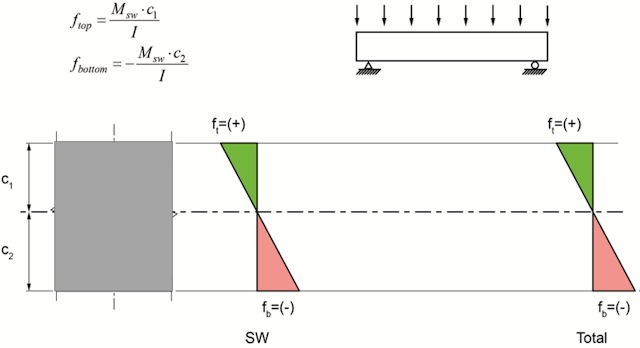### Stress Summaries in a Prestressed Beam

prestressed concrete is advanced technology. The using of prestressed concrete increased recently especially for big structures such as bridge and high rise building. To understand how prestressing of concrete will enhance structure properties. it is useful to draw the stress diagram for a member before and after prestressing.

1. Simply supported beam with self-weight

for a simply supported beam subjected to selfweight load only. The bending stress can be calculated using elastic beam theory f=M.C/I.  the bottom fiber length under self-weight load will increase and this will cause tensile stress as shown in the figure below. the opposite will occur at top fiber. at top fiber, the length will decrease and this will result in compression. concrete is stronger at compression. so the bottom of the member will crack and fail if the load increase beyond the allowable limit.2. Simply supported beam with self weight and axial prestressing force

To enhance the member and reduce the tension at bottom fiber. we will apply a prestressing force PT at the center of the member. this will cause uniform axial stress equal to PT/A as showing in the figure below. as a result, the compression will be reduced at bottom fiber as shown in the figure below.3. Simply supported beam with self-weight and axial prestressing force with eccentricity

To enhance the effects of presstressing force. we will apply PT at eccentricity equal to e. as a result the prestressing force will cause uniform axial stress equal to PT/A. in addition to the axial stress. applying PT at e will cause bending stress (in compression) at bottom fiber and bending stress(in tension) at top fiber as shown in the figure below. as a result, the tension at bottom fiber will be reduced also the compression at top fiber will be reduced. this will result in a member with higher strength compared to previous cases